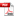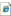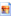# CalculationsNegative Number Introductions

Sets of simple questions of gently increasing complexity for a natural introduction to the wonders of negative numbers.Manipulating Decimals

"Unfortunately the decimal point button is missing from this calculator. Show how you could find answers to these calculations without it." Multiplying and dividing questions, 10 of each.Treasure Hunts

An old favourite. Pupils work out the calculations then colour in the corresponding square on the treasure map, the single remaining square is where the treaure is buried. A great way to make pupils do 50 sums! To follow up, ask pupils to create thier own treasure hunts for you to copy and friends to complete. Question sheets on pages 1, 2, 3. An example of pupils work on page 4. Answers on pages 5, 6, 7.Mental Arithmetic Practice

An excel file for generating endless mental arithmetic practice. Press F9 to recalculate the questions. Try our Ks3 Mental Tests mini-site also.Numeracy Checkup

Sets of questions on various basic numeracy methods. Includes fractions of, percentages of, simplifying linear algebraic expressions, solving simple equations, decimal addition plus several more. 'How to...' document for students to learn independently.Approximation

Two similar sets of questions for pupils to practise calculations after finding upper and lower bounds. Answers to come soon. PDF here.Estimation

A run through of rounding 'to the nearest', 'to decimal places' and 'to significant figures' with a dash of SMSC thrown in.Using a Calculator

Prompts for essentials to learn in order to ge the most from our calculator. Based on using the Casio FX83.I Can Calc

Calculations to check if you really know how to use your calculator. PDF here.Missing Multiplications

Pupils use knowledge of times tables to complete the missing spaces on the tables. Note that digits 2-9 are used only once along top row & LHS column.BISMAS in Russian

Pupils first translate the russian words into numbers and, having done so, do the Russian BIDMAS calculations. Just simple calculations at first and then more complex ones. Answers are easier to mark if given in figures, do as russian words as an extension. You'll need all of these files Russian Numbers , Cryllic and Russian Numbers 2. Don't worry if your Russian isn't good - answers are included!3 Digit Random Number Generator & Countdown Clock!

Does what it says! You can click between two screens, with the number big or small, to provide room to scribble things down on your IWB.

Great for using when playing that popular countdown game. For a 30 second version of the countdown clock music (513Kb mp3) or for a 60 second version (981Kb mp3).

To peice the separate bits of the countdown clock together yourself click 1, 2, 3, 4, 5, 6, 7. Alternatively load them into a sampler and do it 'on the fly'. (But this is only for the really brave!)

Whats left to want?... Perhaps a program to work out the answers?!

For more Countdown stuff than you could ever need see this website!Two Numbers

Sums, differences, products & quotients. Pupils find two numbers that satisfy that calculations. Answers included.Bingo

MS Excel page with a 5x5 bingo style sheet of categories for pupils to make thier own bingo cards for which you then call out the numbers (as mathematical clues). Revises types of number, factors etc & gets all pupils engaged. Can be changed very easily to aid differentiation (e.g. 'multiple of 25' can become 'multiple of 27', can be changed to a 4x4 grid easily...).Times Table Challenge

Pupils race against the clock to complete 10 multiplicationsNon Calculator Number Questions

Worksheet of various number questions. Includes answers.Two Numbers Multiply to 4095

Simple question in MS Word to make pupils consider their logical thinking & mathematical reasoning skills. "Two numbers between 50-70 multiply to make 4095, what are they?". Has worked well with all year groups from Yr7 to Yr12!Maths Puzzle

A good deduction based puzzle for pupils of many ages.Maths Puzzle 2

A shakedown of understanding of column addition methods, BIDMAS, substitution, factors, multiples... leading to an SMSC consideration of the relative frequencies of letters used in the english language.

 Smartboard file starterLesson planKLO'sGraph of letter frequencies by frequencyGraph of letter frequencies by letter